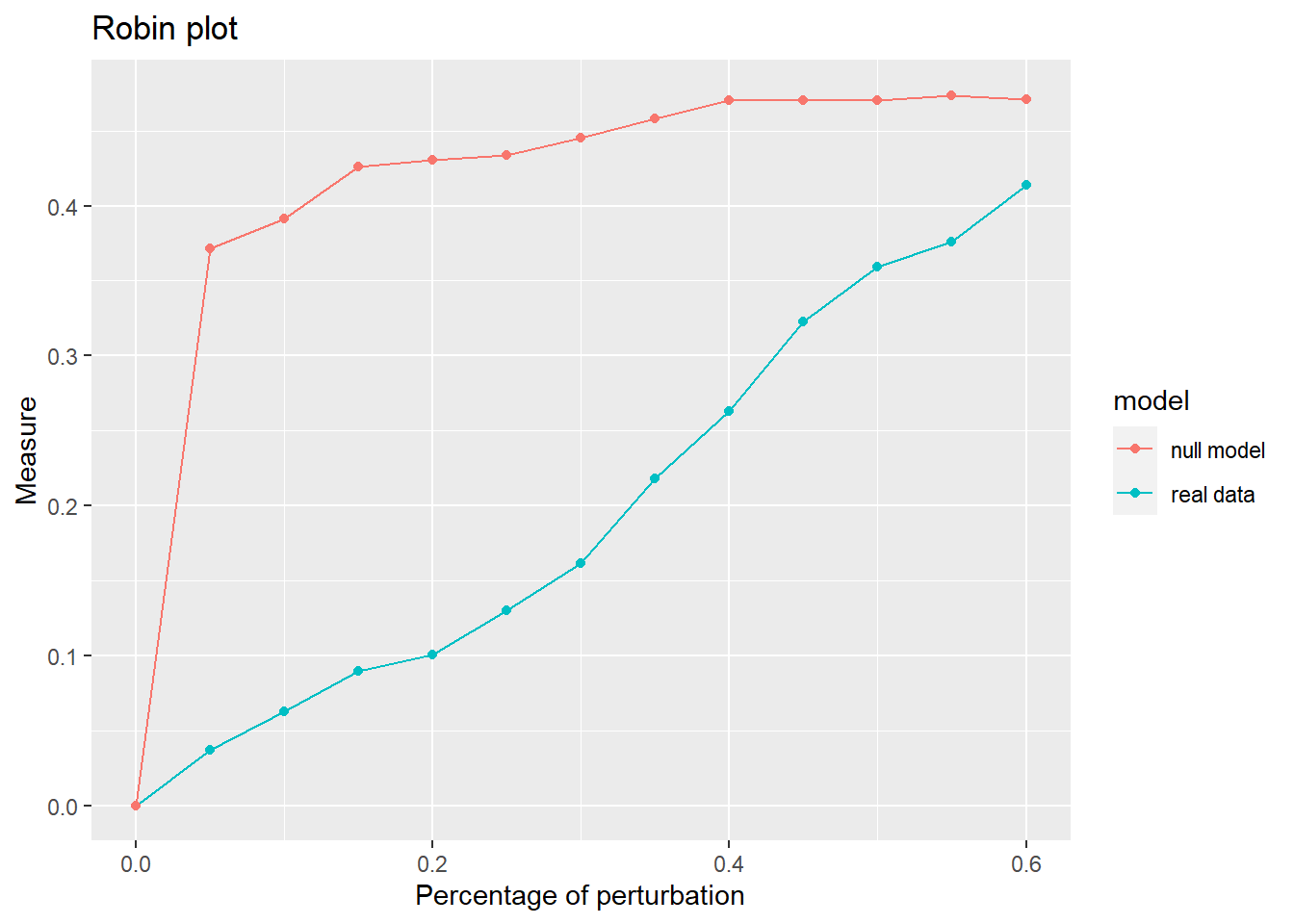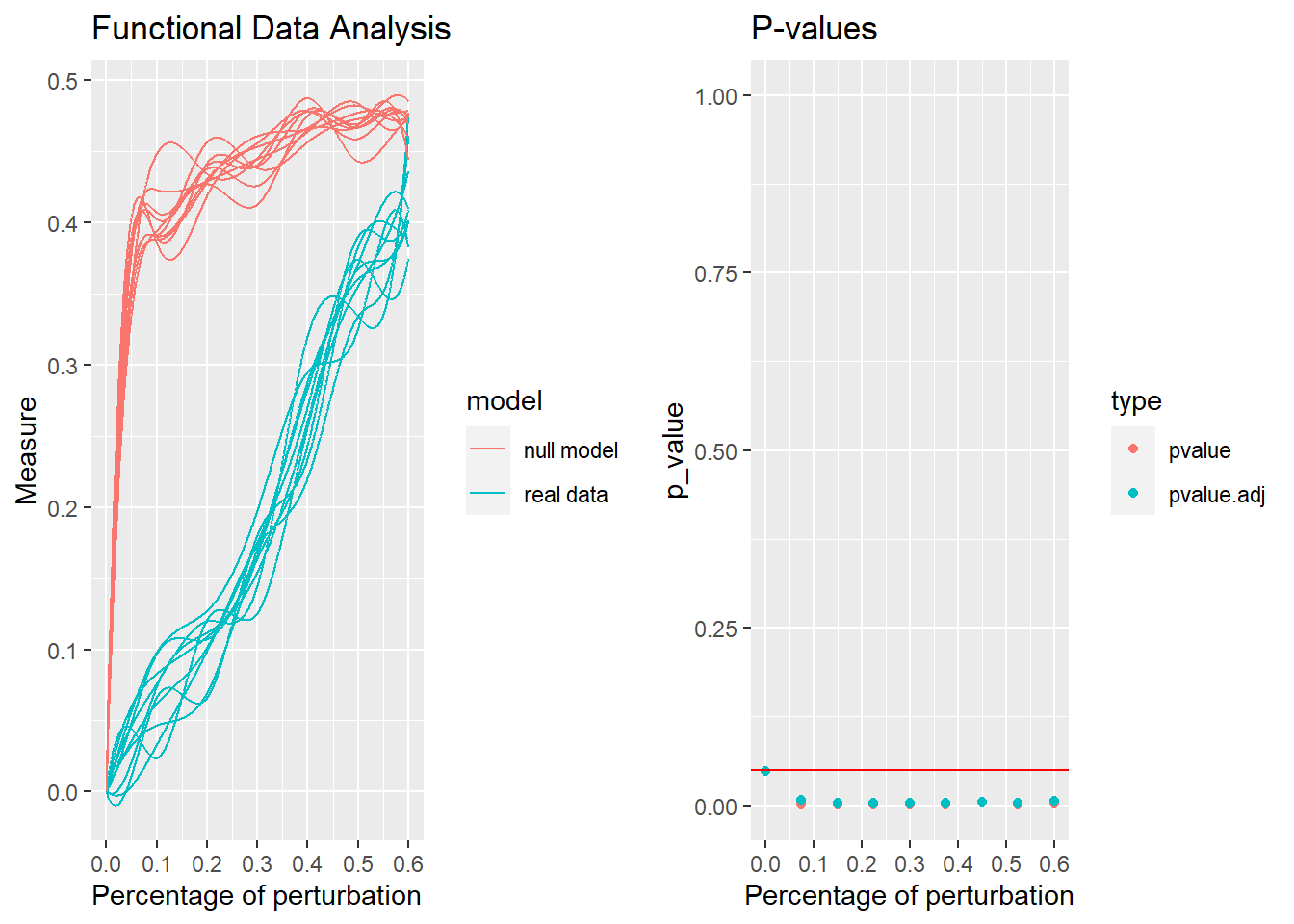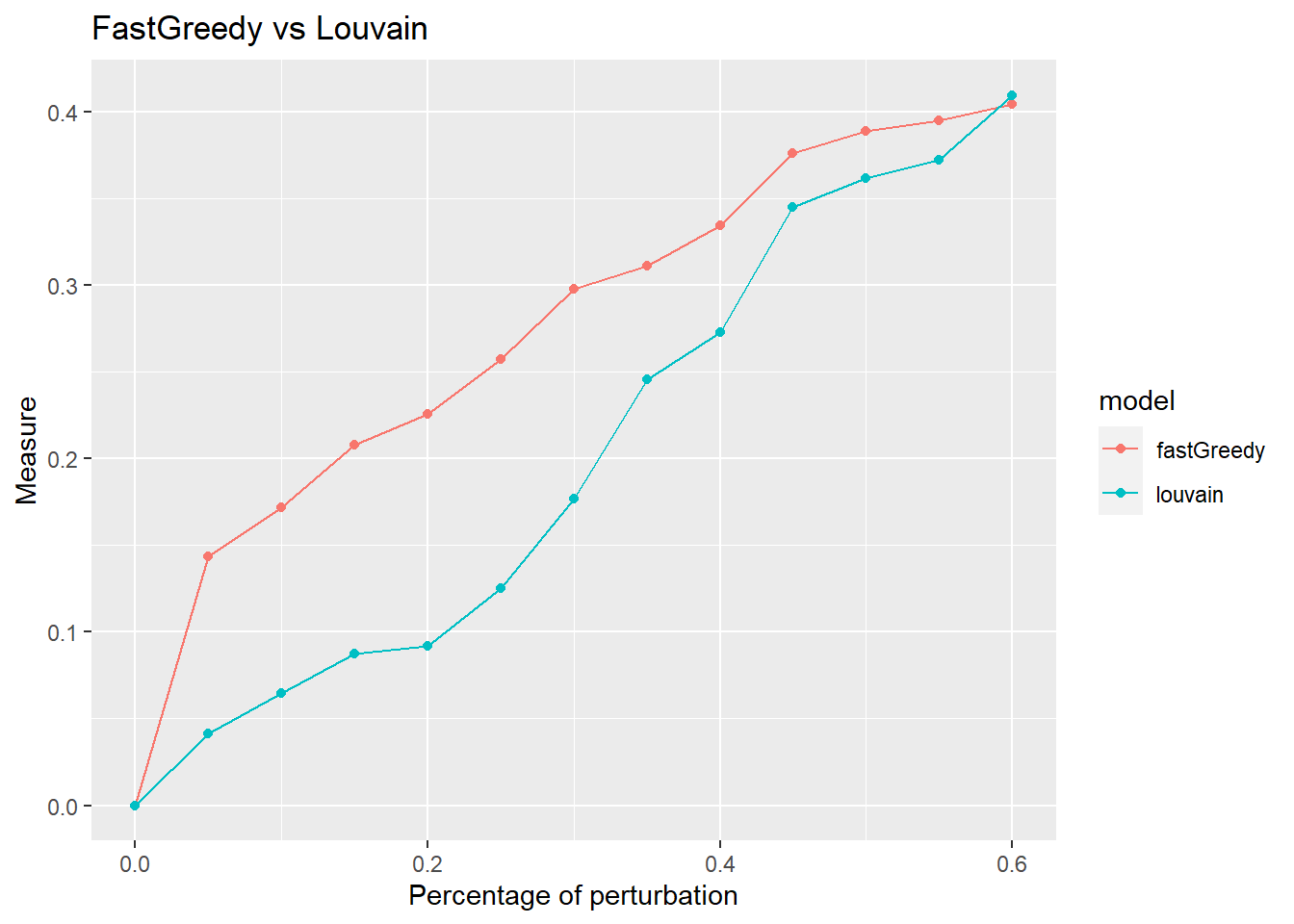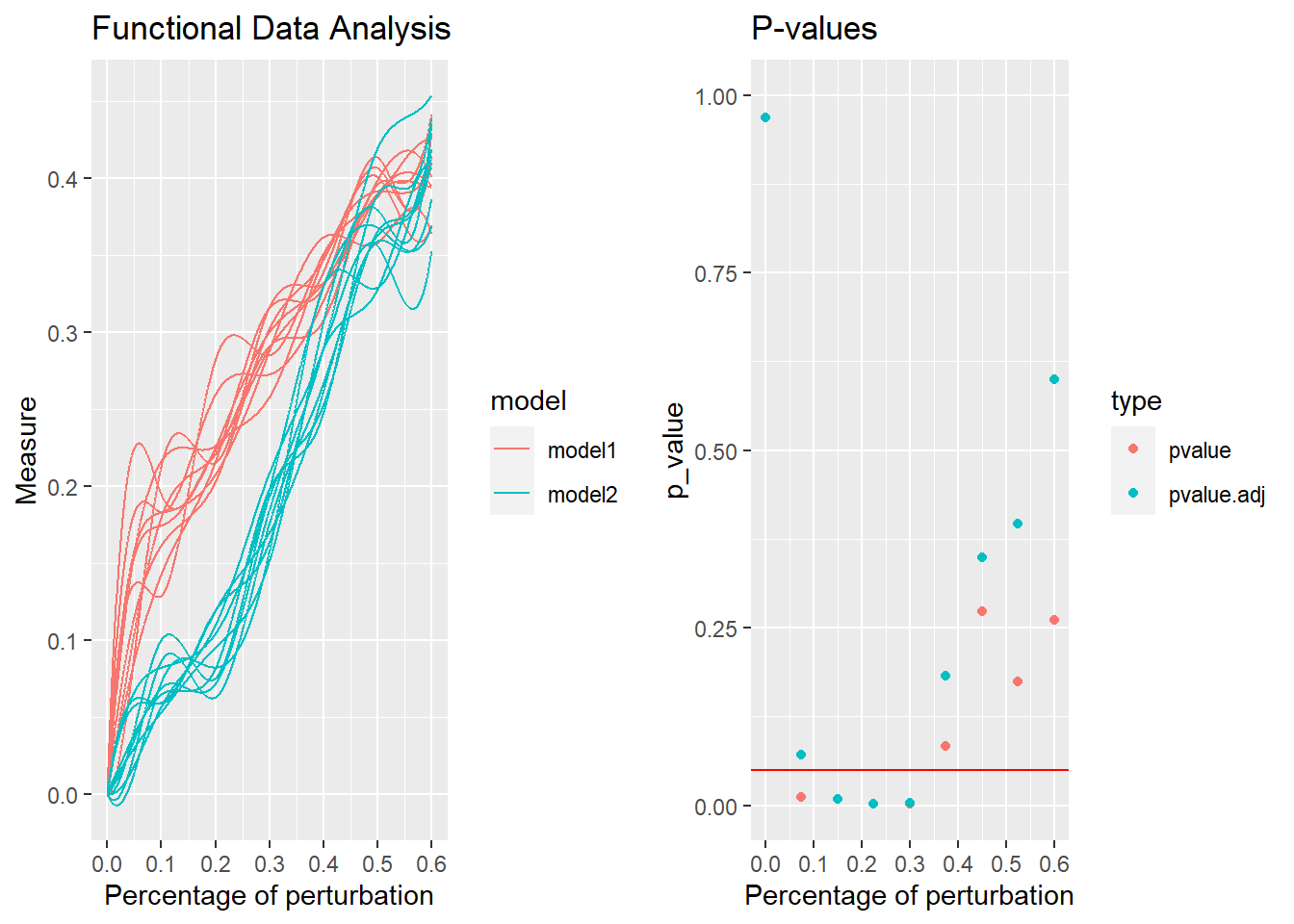# robin

In network analysis, many community detection algorithms have been developed. However,their applications leave unaddressed one important question: the statistical validation of the results.

robin (ROBustness in Network) is an R package for the validation of community detection. It has a double aim: to study the robustness of a community detection algorithm and to compare the robustness of different community detection algorithms on the same network.

It provides: 1) a procedure to examine the robustness of a community detection algorithm against random perturbations of the original graph; 2) two tests to determine the statistical difference between stability measure curves; 3) a routine to choose among different community detection algorithms the one that better fits the network of interest; 4) a graphical interactive representation.

library("robin")

# Input

The input of the robin package is a network that can be read from different format: edgelist, pajek, graphml, gml, ncol, lgl, dimacs, graphdb and igraph graphs.

prepGraph function creates an igraph object from the input file. This step is necessary for robin execution

my_network <- system.file("example/football.gml", package="robin")
graph <- prepGraph(file=my_network, file.format="gml")
graph
## IGRAPH 3181125 U--- 115 613 --
## + attr: id (v/n), label (v/c), value (v/n)
## + edges from 3181125:
##   1--  2 1--  5 1-- 10 1-- 17 1-- 24 1-- 34 1-- 36 1-- 42 1-- 66 1-- 91
##  1-- 94 1--105 2-- 26 2-- 28 2-- 34 2-- 38 2-- 46 2-- 58 2-- 90 2--102
##  2--104 2--106 2--110 3--  4 3--  7 3-- 14 3-- 15 3-- 16 3-- 48 3-- 61
##  3-- 65 3-- 73 3-- 75 3--101 3--107 4--  6 4-- 12 4-- 27 4-- 41 4-- 53
##  4-- 59 4-- 73 4-- 75 4-- 82 4-- 85 4--103 5--  6 5-- 10 5-- 17 5-- 24
##  5-- 29 5-- 42 5-- 70 5-- 94 5--105 5--109 6-- 11 6-- 12 6-- 53 6-- 75
##  6-- 82 6-- 85 6-- 91 6-- 98 6-- 99 6--108 7--  8 7-- 33 7-- 40 7-- 48
##  7-- 56 7-- 59 7-- 61 7-- 65 7-- 86 7--101 7--107 8--  9 8-- 22 8-- 23
## + ... omitted several edges

# Null model

robin offers two choices for the null model:

1. external building according to users’ preferences, then the null graph is passed as a variable;

2. generation by using the function random.

random function creates the null model for the robustness procedure robinRobust. The graph argument must be the same returned by prepGraph function.

The function random creates a random graph with the same degree distribution of the original graph, but with completely random edges, using the rewire function of the package igraph with the option “keeping_degseq” that preserves the degree distribution of the original network. The function rewire randomly assigns a number of edges between vertices with the given degree distribution.

graphRandom <- random(graph=graph)
graphRandom
## IGRAPH 31872c8 U--- 115 613 --
## + attr: id (v/n), label (v/c), value (v/n)
## + edges from 31872c8:
##     2-- 11   1--  5   3-- 10  17-- 62  24-- 78   1-- 34   1-- 36   1-- 92
##    41-- 66  38-- 40  44-- 74  58-- 63   2--108   2-- 65   2-- 34   2-- 38
##    9-- 69  47-- 58  39-- 90   2-- 19  19--103   2--113  67--110   3--  4
##   12-- 61   3-- 76  22-- 58   3-- 97  48-- 81   3-- 61   3-- 28   3-- 50
##   42-- 75   3--106  22--111   4--  6  85-- 91   4-- 84   4-- 88   6-- 51
##    4-- 59  85--111  22-- 71  69-- 82   4--110  26-- 31  14-- 68  10-- 70
##    5--103 108--114  29-- 86  36-- 42   5-- 22   5--110   5-- 78   4--  5
##    6-- 53   6--  9  53-- 70   6-- 89  62-- 82   6-- 17   6-- 91   6-- 75
## + ... omitted several edges

# Network visualization

plotGraph function offers a graphical representation of the network with the aid of networkD3 package.

plotGraph(graph)

## Community detection

methodCommunity function detects communities using all the algorithms implemented in igraph package: “walktrap”, “edgeBetweenness”, “fastGreedy”, “spinglass”, “leadingEigen”, “labelProp”, “infomap”, “optimal”, “other”.

methodCommunity(graph=graph, method="fastGreedy") 
## IGRAPH clustering fast greedy, groups: 6, mod: 0.55
## + groups:
##   $1 ##  7 14 16 33 40 48 61 65 101 107 ## ##$2
##       8   9  10  17  22  23  24  42  47  50  52  54  68  69  74  78  79  89
##    105 109 111 112 115
##
##   $3 ##  1 2 20 26 30 31 34 36 38 46 56 80 81 83 90 94 95 102 ##  104 106 110 ## + ... omitted several groups/vertices membershipCommunities function detects the community membership. membershipCommunities(graph=graph, method="fastGreedy")  ##  3 3 5 5 5 5 1 2 2 2 5 5 4 1 4 1 2 6 4 3 6 2 2 2 5 3 4 6 5 3 3 4 1 3 4 3 6 ##  3 4 1 5 2 6 4 6 3 2 1 6 2 5 2 5 2 4 3 6 6 6 6 1 4 6 6 1 6 6 2 2 5 6 4 5 2 ##  5 6 6 2 2 3 3 5 3 5 5 4 6 6 2 3 5 6 6 3 3 6 6 6 5 4 1 3 5 3 2 3 1 5 2 3 2 ##  2 6 6 2 ## Community visualization plotComm function produces an interactive 3D plot of the communites detected by the chosen algorithm. members <- membershipCommunities(graph=graph, method="fastGreedy") plotComm(graph=graph, members=members) # Robustness of a community detection algorithm robinRobust function implements the validation of the community robustness. In this example we use “vi” distance as stability measure, “indipendent” type procedure and “louvain” as community detection algorithm. Users can choose also different measures as: “nmi”,“split.join”, “adjusted.rand”. proc <- robinRobust(graph=graph, graphRandom=graphRandom, measure="vi", method="louvain", type="independent") plotRobin function plots the stability measure curves. The (x,y)-axes represent the percentuage of perturbation and the average of the stability measure, respectively. plotRobin(graph=graph, model1=proc$Mean, model2=proc$MeanRandom, measure="vi", legend=c("real data", "null model"))The procedure implemented depends on the network of interest.In this example, the Louvain algorithm fits good the network of interest,as the curve of the stability measure assumes lower values than the one obtained by the null model. ## Statistical tests The differeces between the stability measure curves are tested using: 1) Functional Data Analysis (FDA); 2) Gaussian Process (GP). Moreover to quantify the differences between the curves when they are very close the Area Under the Curves (AUC) are evaluated. robinFDATest function implements the FDA testing. robinFDATest(graph=graph, model1=proc$Mean, model2=proc$MeanRandom, measure="vi",legend=c("real data", "null model")) ##  "First step: basis expansion" ## Swapping 'y' and 'argvals', because 'y' is simpler, ## and 'argvals' should be; now dim(argvals) = 13 ; dim(y) = 13 x 20 ##  "Second step: joint univariate tests" ##  "Third step: interval-wise combination and correction" ##  "creating the p-value matrix: end of row 2 out of 9" ##  "creating the p-value matrix: end of row 3 out of 9" ##  "creating the p-value matrix: end of row 4 out of 9" ##  "creating the p-value matrix: end of row 5 out of 9" ##  "creating the p-value matrix: end of row 6 out of 9" ##  "creating the p-value matrix: end of row 7 out of 9" ##  "creating the p-value matrix: end of row 8 out of 9" ##  "creating the p-value matrix: end of row 9 out of 9" ##  "Interval Testing Procedure completed"## TableGrob (1 x 2) "arrange": 2 grobs ## z cells name grob ## 1 1 (1-1,1-1) arrange gtable[layout] ## 2 2 (1-1,2-2) arrange gtable[layout] ##$adj.pvalue
##  0.0482 0.0075 0.0033 0.0028 0.0028 0.0029 0.0047 0.0028 0.0060
##
## $pvalues ##  0.0482 0.0023 0.0023 0.0023 0.0023 0.0023 0.0047 0.0023 0.0040 The first figure represents the stability measure plot using “louvain” algorithm for detecting communities. The second one represents the corresponding adjusted p-values of the Interval Testing procedure. Horizontal red line corresponds to the critical value 0.05. robinGPTest function implements the GP testing. robinGPTest(model1=proc$Mean, model2=proc$MeanRandom) ## Profile 1 ## Profile 2 ##  105.6061 The output is the Bayes Factor. robinAUC function implements the AUC. robinAUC(graph=graph, model1=proc$Mean, model2=proc$MeanRandom, measure="vi") ##$area1
##  0.116362
##
## $area2 ##  0.2557771 The outputs are the area under the two curves # Comparison of two community detection algorithms robinCompare function compares two detection algorithms on the same network and permits the user to choose the one that better fits the network of interest. In this example we consider the “Fast Greedy” and “Louvain” algorithms. We firstly plot the communities dectected by both algorithms. membersFast <- membershipCommunities(graph=graph, method="fastGreedy") membersLouv <- membershipCommunities(graph=graph, method="louvain") plotComm(graph=graph, members=membersFast) plotComm(graph=graph, members=membersLouv) Secondly, we compare them with robinCompare function. comp <- robinCompare(graph=graph, method1="fastGreedy", method2="louvain", measure="vi", type="independent") Thirdly, we plot the curves of the compared methods. plotRobin(graph=graph, model1=comp$Mean1, model2=comp$Mean2, measure="vi", legend=c("fastGreedy", "louvain"), title="FastGreedy vs Louvain")In this example, the Louvain algorithm fits better the network of interest, as the curve of the stability measure assumes lower values than the one obtained by the Fast greedy method. ## Statistical tests The following procedures test the statistical differences between the two curves using two different methods robinFDATest(graph=graph, model1=comp$Mean1, model2=comp$Mean2, measure="vi") ##  "First step: basis expansion" ## Swapping 'y' and 'argvals', because 'y' is simpler, ## and 'argvals' should be; now dim(argvals) = 13 ; dim(y) = 13 x 20 ##  "Second step: joint univariate tests" ##  "Third step: interval-wise combination and correction" ##  "creating the p-value matrix: end of row 2 out of 9" ##  "creating the p-value matrix: end of row 3 out of 9" ##  "creating the p-value matrix: end of row 4 out of 9" ##  "creating the p-value matrix: end of row 5 out of 9" ##  "creating the p-value matrix: end of row 6 out of 9" ##  "creating the p-value matrix: end of row 7 out of 9" ##  "creating the p-value matrix: end of row 8 out of 9" ##  "creating the p-value matrix: end of row 9 out of 9" ##  "Interval Testing Procedure completed"## TableGrob (1 x 2) "arrange": 2 grobs ## z cells name grob ## 1 1 (1-1,1-1) arrange gtable[layout] ## 2 2 (1-1,2-2) arrange gtable[layout] ##$adj.pvalue
##  0.9683 0.0708 0.0089 0.0019 0.0037 0.1824 0.3494 0.3967 0.6003
##
## $pvalues ##  0.9683 0.0113 0.0089 0.0016 0.0016 0.0838 0.2736 0.1745 0.2603 robinGPTest(model1=comp$Mean1, model2=comp$Mean2) ## Profile 1 ## Profile 2 ##  65.77978 while robinAUC(graph=graph, model1=comp$Mean1, model2=comp$Mean2, measure="vi") ##$area1
##  0.1662205
##
## \$area2
##  0.1194338

calculates the area under the curves.